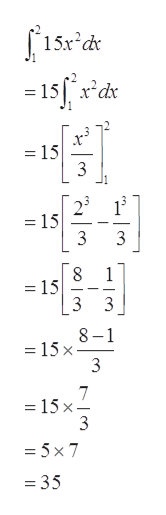Question
14 views
check_circle

Step 1

Given:

Step 2

Concept used:

Fundamental Theorem of calculus states that if f is continuous on the closed interval [a, b] and F is the indefinite integral of f on [a, b] then

Step 3

Calculate the integration with respect to ‘x’...help_outlineImage Transcriptionclose= 15f r'dx = 15 3 2 1 = 15 3 3 8. = 15 3 3 8-1 = 15 x 3 = 15 x- 3 = 5x 7 = 35 fullscreen

### Want to see the full answer?

See Solution

#### Want to see this answer and more?

Solutions are written by subject experts who are available 24/7. Questions are typically answered within 1 hour.*

See Solution
*Response times may vary by subject and question.
Tagged in

### Integration